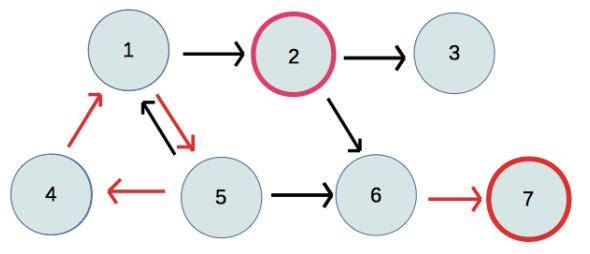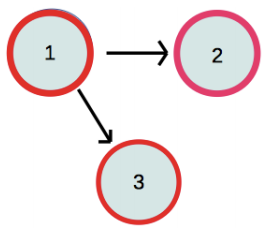시간 제한메모리 제한제출정답맞힌 사람정답 비율
2 초 512 MB14121083.333%

## 문제

As part of a CS course, Alice just finished programming her robot to explore a graph having n nodes, labeled 1, 2, . . . , n, and m directed edges. Initially the robot starts at node 1.

While nodes may have several outgoing edges, Alice programmed the robot so that any node may have a forced move to a specific one of its neighbors. For example, it may be that node 5 has outgoing edges to neighbors 1, 4, and 6 but that Alice programs the robot so that if it leaves 5 it must go to neighbor 4.

If operating correctly, the robot will always follow forced moves away from a node, and if reaching a node that does not have a forced move, the robot stops. Unfortunately, the robot is a bit buggy, and it might violate those rules and move to a randomly chosen neighbor of a node (whether or not there had been a designated forced move from that node). However, such a bug will occur at most once (and might never happen).

Alice is having trouble debugging the robot, and would like your help to determine what are the possible nodes where the robot could stop and not move again.

We consider two sample graphs, as given in Figures G.1 and G.2. In these figures, a red arrow indicate an edge corresponding to a forced move, while black arrows indicate edges to other neighbors. The circle around a node is red if it is a possible stopping node.Figure G.1: First sample graph. Figure G.2: Second sample graph.

In the first example, the robot will cycle forever through nodes 1, 5, and 4 if it does not make a buggy move. A bug could cause it to jump from 1 to 2, but that would be the only buggy move, and so it would never move on from there. It might also jump from 5 to 6 and then have a forced move to end at 7.

In the second example, there are no forced moves, so the robot would stay at 1 without any buggy moves. It might also make a buggy move from 1 to either 2 or 3, after which it would stop.

## 입력

The first line contains two integers n and m, designating the number of nodes and number of edges such that 1 ≤ n ≤ 103, 0 ≤ m ≤ 104. The next m lines will each have two integers a and b, 1 ≤ |a|, b ≤ n and |a| ≠ b. If a > 0, there is a directed edge between nodes a and b that is not forced. If a < 0, then there is a forced directed edge from −a to b. There will be at most 900 such forced moves. No two directed edges will be the same. No two starting nodes for forced moves will be the same.

## 출력

Display the number of nodes at which the robot might come to a rest.

## 예제 입력 1

7 9
1 2
2 3
-1 5
2 6
5 1
-4 1
5 6
-6 7
-5 4


## 예제 출력 1

2


## 예제 입력 2

3 2
1 2
1 3


## 예제 출력 2

3